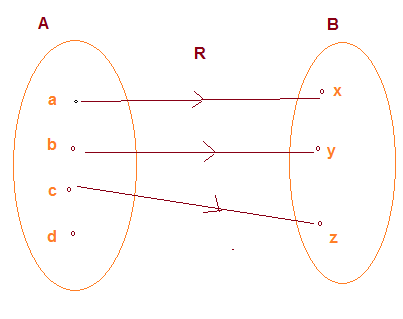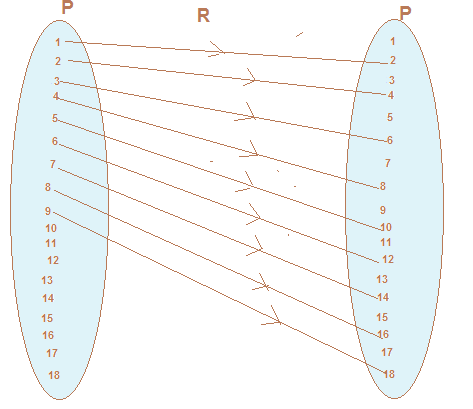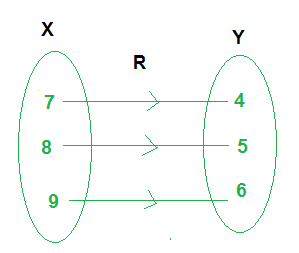f Relation in Maths | Definition | Pictorial Representation# Relation in Maths

## 2. What is relation?

Definition: A relation $R$ from a non-empty set $A$ to a non-empty set $B$ is a subset of the Cartesian product $A \times B$.
It "maps" elements of one set to another set. The subset is derived by describing a relationship between the first element and the second element of the ordered pair $\left( {A \times B} \right)$.
Domain: The set of all first elements of the ordered pairs in a relation $R$ from a set $A$ to a set $B$ is called the domain of the relation $R$.
Range: the set of all the ending points is called the range
Co-domain: The whole set B is called the co-domain of the relation R.
Example
Let A ={4,5,3} and B ={1,6, 7}
Let R be the Relation "is greater than " from A to B
Then
R= { (4,1),(5,1),(3,1)}

## Algebraic Representation of Relation

A relation can be expressed in Set builder or Roaster form

### Roster forms

In a Roster forms, all the ordered pair in the relation is listed.
Example
R= { (4,1),(5,1),(3,1)}

#### Some Important points

• In roster form, the order in which the elements are listed is immaterial
• while writing the set in roster form an element is not generally repeated

### Set Builder Form

• In set-builder form, all the ordered pair of a relation possess a single common property which is not possessed by any ordered pair outside the relation. For example, in the relation $\left\{ (1,2) ,(2,4) ,(3,6) ,(4,8),(5,10) \right\}$, all the ordered pairs possess a common property, namely, second element in ordered is doubled of first element . Denoting this set by $R$, we write
$R = \left\{(x,y) : x,y \in {1,2,3,4,5,6,7,8,9,10},y=2x \right\}$

## Pictorial Representation of Relation

A Relation R from A to B can be depicted pictorially using arrow diagram . In arrow diagram, we write down the elements of two set A and B in two disjoint circle,Then we draw arrow from set A to set B whenever $(a,b) \in R$

Let A={a,b,c,d} and B={x,y,z}
And R ={(a,x),(b,y),(c,z)}
Then this will be represented in arrow diagram as#### Important Note

The total number of relations that can be defined from a set $A$ to a set $B$ is the number of possible subsets of $A \cdot B$. If $n\left( A \right) = p$ and $n\left( B \right) = q$, then $n\left( {A \cdot B} \right) = pq$ and the total number of relations is ${2^{pq}}$

Example:
Let $P = \left\{ {1,2,3,.....,18} \right\}$ define a relation $R$ from $P$ to $P$ by $R = \left\{ {\left( {x,y} \right):2x - y = 0,where \; x,y \in P} \right\}$ Write down its domain, co-domain and range.
Draw the arrow diagram for the relation also
Solution: The relation $R$ from $P$ to $P$ is given as
R = {(x,y):2x-y=0, where x, y ∈ P}
i.e., R = {(x, y): 2x = y, where x, y ∈ P}
Therefore,
$R = \left\{ {\left( {1,2} \right),\left( {2,4} \right),\left( {3,6} \right),\left( {4,8} \right),\left( {5,10} \right),\left( {6,12} \right),\left( {7,14} \right),\left( {8,16} \right),\left( {9,18} \right)} \right\}$
The domain of $R$ is the set of all first elements of the ordered pairs in the relation.
Therefore,
$Domain \; of \; R = \left\{ {1,2,3,4,5,6,7,8,9} \right\}$
The whole set $P$ is the co-domain of the relation $R$.
Therefore co-domain of $R = P = \left\{ {1,2,3, \ldots ,18} \right\}$
The range of $R$ is the set of all second elements of the ordered pairs in the relation.
Therefore range of $R = \left\{ {2,4,6,8,10,12,14,16,18} \right\}$
Arrow diagram is given below### Quiz Time

Question 1.
Let P ={a,b,c} and Q={x,y,z} then which is of the below is not a relation from P to Q
A. R = {(a,x),(b,y),(c,z)}
B. R = {(a,y),(b,z), (c,x)}
C. R = {(x,a),(b,y),(c,z)}
D. None of the above
Question 2.
Let A = {1, 2, 3,4} and B = {5, 7}. Then possible number of relation from A to B ? A. 64
B. 256
C. 16
D. 32
Question 3.
A = {1, 2, 3, 5} and B = {4, 6, 9}. Define a relation R from A to B by $R = \left \{ (x, y): the \; difference \; between \; x \; and \; y \; is \; odd; x \in A, y \in B \right \}$. R is roster form is given by
A. $R ={(1,4),(1,6),(2,9),(3,4),(3,6),(5,4),(5,6)}$
B. $R ={(1,4),(1,6),(2,9),(3,4),(3,6),(5,6)}$
C. $R ={(1,4),(1,6),(2,9),(3,4),(3,6)}$
D. $R ={(1,4),(1,6),(2,9),(3,4),(3,6),(4,5),(5,6)}$
Question 4.
Let A = {1, 2, 3,4, 5,6,7,8,9} and R is relation defined on Set A as R ={(1,1),(2,4),(3,9)}. What is the range of the relation?
A. {1,2,3,4,5,6,7,8,9}
B. {1,2,4,3,9}
C. {1,4,9}
D. {1.2.3}
Question 5.
What is the domain in the above relation
A. {1,2,3,4,5,6,7,8,9}
B. {1,2,4,3,9}
C. {1,4,9}
D. {1.2.3}
Question 6.
Check the below arrow diagram and find out the relation in roster formA. R={(7,4),(8,5),(9,6)}
B. R={(4,7),(5,8),(6,9)}
C. R={(7,3),(8,3),(9,3)}
D. R={(3,4),(3,5),(3,6)}